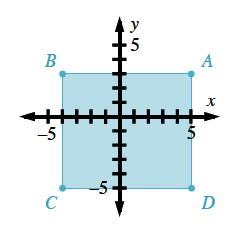### Home > AC > Chapter 2 > Lesson 2.1.3 > Problem2-31

2-31.

Plot the points A$(5,3)$, B$(−4,3)$, C$(−4,−6)$, and D$(5,−6)$ on a set of axes. Use a ruler to connect them in order, including D back to A, to form a quadrilateral (a shape with four sides).

1. What kind of quadrilateral was formed?All of the sides are the same length; therefore, what shape is it?

A square

1. How long is each side of the quadrilateral?

Count the distance between each corner.

1. What is the area of the quadrilateral?

Multiply the width by the length.

1. What is the perimeter of the quadrilateral?

Add up all of the lengths of the sides.